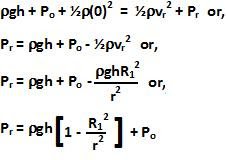## Saturday, May 9, 2009

### Irodov Problem 1.321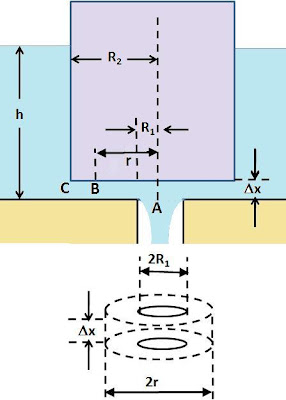The total pressure at point C, at the bottom of the cylinder is given by, where h is the height of the water level and Po is the atmospheric pressure. At point C, the water is assumed to be still and so its velocity is 0. At point A, the water freely falls and the only pressure is once again the atmospheric pressure Po. Let the velocity of the water immediately after the orifice be v. Then, applying Bernoulli's equation for points C and A, we have,The amount of water flowing per unit time at any point in the orifice can be determined by multiplying the surface area of section through which the water times the velocity of the water.

Near point A, the water powers through a cylindrical section of height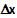and radius R1. In others words the total volume of water flowing out per unit time is given by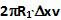.

consider a point B within the orifice that is t a radial distance r from the central axis of the cylinder. The surface area of the section through which water is flowing at this point is given by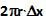. Let the velocity of the water at point B be vr. Since the total amount of water flowing through any cross-section per unit time must be the same, we have,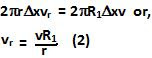Let the internal pressure of the fluid at point B be Pr. Then applying Bernoullis equation for points A and B we have,